# Community

Blog Events Webinars Tutorials Forum
×
Community Blog Deep Dive into Understand Bayesian Cclassification Aalgorithm

# Deep Dive into Understand Bayesian Cclassification Aalgorithm

A lot of our online experience depends significantly on machine learning algorithms. One such algorithm is Bayesian Classification Algorithm for examp.

## 1. Introduction:

Bayesian classification is the general term for a type of classification algorithm based on Bayes theorem. This article will start by introducing the classification problem and will then give its definition. Then we will introduce Bayesian theorem as it pertains to the Bayes classification algorithm. Finally, we will discuss the simplest type of Bayesian classification - naive Bayesian classification - and explain it along with application cases.

## 2. Bayesian Classification

### 2.1 Summary - The Issue of Classification

Most of us are already familiar with the concept of classification as we, more or less, use them every day. For example, when you see a stranger, your brain subconsciously determines that this person is either a man or a woman. In fact, this is a sort of classification operation.

From a mathematical standpoint, we can define classification problems as follows:

Already Known Sets: {C=y1,y2,...,yn}and I={x1,x2,...,xm,...}, determine the mapping rules y=f(x), 0 is arbitrarily defined as any xi∈I, and only one item yi∈C is yi=f(xi)true.

Where C is called a collection of categories, each element is a category, and I is the name by which the item set is called with each element an item to be sorted, and f is called a classifier. The task of the classification algorithm is to construct the classifier f.

We will emphasize here that classification problems often use empirical methods to construct mapping rules. That is to say; general classification problems frequently lack enough information to construct a 100% correct mapping rule. However, through the experience of data learning, it is possible to achieve a certain probability of getting the correct classification. Therefore, the trained classifier does not necessarily need to accurately map each item to its classification. The quality of the classifier is related to the classification construction method, the characteristics of the classifiable data, and the number of samples provided.

For example, a doctor's diagnosis of a patient is a typical sort of classification process. No doctor can directly see the patient's condition, he or she can only observe the patient's visible symptoms and use various test data to infer the likely conditions. Therefore, we can see that the doctor is a classifier and that the doctor's diagnosis accuracy rate is closely related to:

•The method of his/her education (structured),
•Whether the patient's symptoms were prominent (the characteristics of the data being categorized), and
•How much experience the doctor has had (the number of training samples).

### 2.2 Bayesian Classification Based on Bayes' Theorem

Bayes' Theorem solves many problems that we often encounter in real life. We can generalize most of these problems as follows:

"Given that, an event has occurred (by assumption, presumption, assertion or evidence) what is the probability that a second event will occur?"

We will express the already known condition as P(A|B) and the event which is likely to potentially occur as P(B|A). We will begin by explaining what conditional probability is:

P(A|B) indicates that event B has already occurred. The probability of event A is called the conditional probability of event A in event B. The basic solution formula is: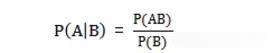Bayes' Theorem is useful because we often encounter this sort of thing in our daily lives: we can easily draw a direct conclusion P(A|B), but P(B|A) is harder to infer directly. However, we're more concerned about P(B|A). Bayes Theorem is the path that lets us travel from P(A|B) to P(B|A).
The below is Bayes' Theorem without proofs: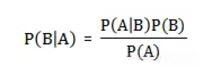### 2.3 Naive Bayesian classification: Principles and Processes

Naive Bayes (classifier) is a type of generative model that models each possible category based on training samples. We call it as "Naive Bayes" because of the assumption that each attribute has conditional independence. This assumes that each attribute has an independent effect on the eventual classification result. The following formula is available to us: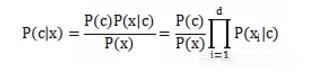The next thing to take particular note of is whether or not a probability value of 0 will affect the subsequent estimations. Therefore, we set a very small value for the probability of an attribute that will not appear. This value is not 0. We call it as Laplacian correction.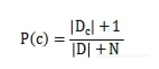Laplacian correction assumes an even distribution of attribute values and categories and introduces additional prior knowledge into the learning process.

2.3.1 Three Stages of the Naive Bayesian Classification

Stage 1: Preparatory Work Stage
In this stage, we do the necessary preparation for Naive Bayesian classification. The main task is to determine the characteristics of the attributes according to the attributes' specific conditions and to perform the appropriate division of each feature attribute. Then, we select a portion of the classification for sampling and use it to form the training sample set. The input for this stage is all the classifiable data, and the output is the characteristic attributes and training samples. This stage is the only stage in the whole of naive Bayesian classification where the quality of the work performed will have a heavy influence on the whole process. The characteristics of the attributes, classification of the characteristic attributes, and the quality of the training samples decide the quality of the classifiers.

Stage 2: Classifier Training Stage
In this stage, we generate a classifier. The main task is to calculate the frequency of appearance of each category in the training samples and the conditional probability estimation of each category for each feature attribute and to record the results. The inputs are feature attributes and the training sample; the outputs are classifiers. This stage is a mechanical one; it is based on the formula discussed above, and we can automatically calculate it completely by a program.

Stage 3: Application Stage
The task in this stage is to classify the classification items using the classifier. The inputs are the classifier and the classifiable items, and the output is the mapping between the classifiable items and the categories. This stage is also a mechanical and we can complete it through the program.

### 2.4 Semi-naive Bayesian Classification

In Naive Bayesian Classification, we assumed the independence of the various attributes. We did this for convenience in computation and to prevent an excessively large number of computations caused by the dependence of excessive attributes. Therefore, we call it "naïve." Although the overall effect achieved by native Bayesian classification is not bad, this is possible because the attributes are related to each other. The properties of one attribute depend on the properties of another. Therefore, these are semi-naive Bayesian classifiers.

To limit the size of calculations, we must assume that each attribute relies only on one other attribute. In this way, a more accurate description of the real situation is possible.

The formula becomes: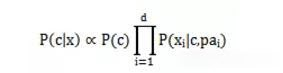pai Attributes for xi the dependent attribute become the xi parent attribute.

We can use following methods to determine the parent attribute:

SOPDE Method: This approach assumes that all attributes depend on a common parent property.
TAN Method: A max weighted spanning tree determines every additional attribute that each attribute depends on
•We first evaluate the mutual information between each attribute as the weight between them.
•The complete graph of the components. Weight is the mutual information just obtained. Then we use the max weighted spanning tree algorithm to get this graph's maximum spanning tree.
•Find a root variable, then turn the diagram into a forward graph.
•Add classification y to the directed edge of each attribute.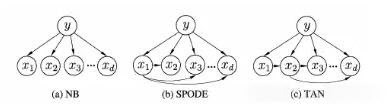Image 1: Three methods' property dependencies

## 3. Application Examples of a Bayesian Algorithm

Here are some practical examples to illustrate the universality of the Bayesian approach. All of the below examples focus on machine learning.

### 3.1 Chinese Word Segmentation

Wu Jun, a researcher at Google, describes in an article in "The Beauty of Mathematics" series how we can do Chinese word segmentation. Here, we will only introduce the basics behind the core of the idea.

Because the Chinese language does not have spaces, there can be considerable difficulty in determining where a word ends and another word begins. For example, in the given sentence/word string - 南京市长江大桥 (Nanjing City Yangtze River Bridge) - there is no clear differentiation. What is the most reliable way to perform segmentation on this word string so that we can parse it correctly? We have the following choices available to us:

•Nanjing City / Yangtze River Bridge
•Nanjing / Mayor / River Bridge

Which one of these choices is more correct?

We use the Bayesian formula to formalize the problem, making X a string (sentence) and Y a word string (a particular hypothesis for correct word segmentation). We just need to find the Y that maximizes P(Y|X):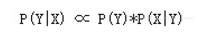To describe this in natural language, we will multiply the possibility of this word segmentation (word string) with the possibility of us generating our sentence by use of this word string. Going to the next step, we can easily see: P(X|Y) approximates equal to 1 because any imaginary way of generating a word under our sentence is always generated accurately (just throw away the demarcation sign between the participles). Therefore, we have achieved the maximization of P(Y) which is to find a word string (sentence) with maximum probability of being correct. How is the word string computed: What is the possibility of W1, W2, W3, W4...?

According to the expansion of the formula of joint probability, we know that: P(W1, W2, W3, W4 ..) = P(W1) P(W2|W1) P(W3|W2, W1) P(W4|W1, W2, W3) .. Therefore we can find the whole joint probability by the product of a series of conditional probabilities (right). Unfortunately, as the number of conditions increases to n-1 conditions P(Wn|Wn-1, Wn-2, .., W1), the problem of sparse data will become progressively more serious. Datasets which are too large also cannot count on getting a reliable P(Wn|Wn-1, Wn-2, .., W1).

To alleviate this problem, many computer scientists always used the "naive" hypothesis. We assume that the probability of a word appearing in a sentence depends only on the preceding words, which is a finite number k. (k usually does not exceed 3, if it relies only on one of the preceding words. This is the 2-gram language model. We can use the same idea for 3-gram and 4-gram models).

This is the so-called "limited horizon" hypothesis. Although it is likely that you will initially find this assumption somewhat idealistic, the results are often very strong. The naive Bayesian method used in the following assumptions is completely consistent with these results. We will explain further why such an idealized hypothesis can lead to powerful results.

So far as we know, with this assumption, we can rewrite the final product as follows: P(W1) P(W2|W1) P(W3|W2) * P(W4|W3)...(Suppose each word relies only on one word in front of it). Statistically, P(W2|W1) will no longer be plagued by the problems of sparse data. Regarding our example mentioned above 南京市长江大桥. If we are reading from right to left, our result is not Nanjing City / Yangtze River Bridge but is, instead, Nanjing / Mayor / River Bridge. However, if we use Bayes to divide the words (assuming the use of the 3-gram model), because Nanjing Mayor and River Bridge have a corpus frequency of 0, the algorithm will judge whole sentence's probability to be 0. As a result, the correct word string Nanjing City / Yangtze River Bridge wins out.

### 3.2 Bayes Spam Filters

For a given email, determine whether or not the system should send it to the spam box. According to precedent, we will still use the variable D to express this given email, note that D is made up of N words. We will use h+ to represent spam and h- to represent normal mail which we want. We can describe the problem formally as the following request:

P(h+|D) = P(h+) * P(D|h+) / P(D)
P(h-|D) = P(h-) * P(D|h-) / P(D)

The two possibilities of P(h+) and P(h-) are easy enough to find, and as a result, we can calculate the proportion of spam to normal messages in an inbox. However, P(D|h+) is not easy to find, because D contains N words d1, d2, d3, and so on. Therefore, P(D|h+) = P(d1, d2, ..., dn|h+) Once again, we have encountered the problem of data sparsity. Why do we say that?

P(d1,d2,..,dn|h+) means that the probability of a given message already in spam being the same as an incoming message is very small. This is because each message is different and there are countless emails constantly coming in. This is data sparsity because it is certain that the training database you use, no matter how many emails it contains, will not be large enough to have incoming mail identical to other incoming mail. The result? How do we compute P (d1, d2, .., dn|h+)?

We extend P (d1, d2, .., dn|h+) to: P(d1|h+) P(D2|D1, h) P(D3|D2,D1, h) ., We certainly are not no familiar to this formula, and here we will use a more radical assumption. We will assume that di is completely unrelated to di-1 and that the equation is reduced to P(d1|h+) P(d2|h+) P(d3|h+) .. This is the so-called conditional independent hypothesis, and it is also the naive Bayesian approach's simplicity. However, when calculating P(d1|h+) P(d2|h+) P(d3|h+) * .. the problem becomes simple enough, as long as the statistical word di frequently appears in previously identified spam.

From studying the above, we discover that Bayesian inference basically cannot give a positive result because of its inability to raise all possibilities. However, after a lot of testing, if the test results are correct, we will also have confidence in our algorithm (even if we have not confirmed the accuracy of the algorithm). In fact, with the new test results, the number of errors produced by the algorithm gradually decreases, and the algorithm becomes more trustworthy.

In our daily lives, we often intentionally or unintentionally make use of the Bayes theorem. From the point of view of the algorithm, if you want to understand the principle, you also need to learn more in-depth mathematical statistics and practices.

## 4. Conclusion

We have seen how Bayesian Classification Algorithm helps us in solving some real-world problems. The applications built on this algorithm can become progressively better with time. Hopefully, you have liked this article. To know more about Alibaba Cloud and its offerings, please visit www.alibabacloud.com

0 0 0
Share on

# Related Products

• ##Global Network Solution

Alibaba Cloud's global infrastructure and cloud-native SD-WAN technology-based solution can help you build a dedicated global network

• ##Networking Overview

Connect your business globally with our stable network anytime anywhere.

• ##Global Application Acceleration Solution

This solution helps you improve and secure network and application access performance.

• ##CloudAP

A new-generation wireless network access product based on cloud and big data platforms.